SEARCH HOMEMath Central Quandaries & QueriesHi there, please, explain me synthetic division of polynomial functions. for examp.: 3x^3 + 2x^2 +4 divide by x-2 (number after variable x is the power of x ) Thank you. Edward.Hi Edward,

Synthetic division is a method of dividing polynomials.
When given 3x3 + 2x2 + 4 divided by x-2, you first must solve for x.

So set x-2=0, then x=2

To use this with synthetic division, we must take the coefficients in the polynomial and make sure all powers of x are accounted for. So, 3x3 + 2x2 + 0x1 + 4 (notice there was no coefficient for x1, so we use 0 as a place holder).

Then we are ready to use synthetic division. The x value goes on the outside the box and the polynomial coefficients inside.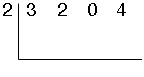We bring the first number down,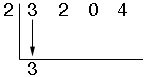then multiply it by our divisor (32 = 6) and place this value under our next coefficient.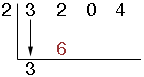Add these 2 numbers (2+6=8). The answer is placed vertically below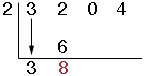and used as our next number to be multiplied by the divisor (82 = 16),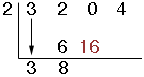continue this process 'till you run out of coefficients.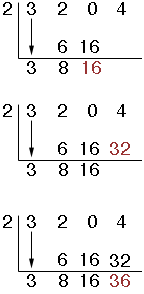The number left over in the bottom right corner is your remainder. The numbers to the left of this are your quotient. (remember we are going down by one power)

ie. (3x3 + 2x2 + 4)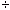(x - 2) = 3x2 + 8x1 + 16 remainder: 36

Hope this helps.
MelanieMath Central is supported by the University of Regina and The Pacific Institute for the Mathematical Sciences.